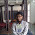## 79xx Series Three Terminal Voltage Regulators

by realfinetime  |  in Voltage Regulator at  06:14

We have already seen 78xx series of positive voltage regulators. Negative voltage regulators are equally important as positive voltage regulators. 79xx series of voltage regulators are the commonly used negative voltage regulators. These are three terminal regulators and is available with fixed output voltages of -5V, -12V and -15V. These ICs have internal current limiting protection and thermal shut down protection to protect the ICs from overload conditions.

Features of 79xx Series of ICs.

1. 1.5A Output Current.
2. High ripple rejection.
3. 4% tolerance on preset output voltage.
4. Thermal, Short circuit and safe area protection.

Pinout Diagram of 79xx Series ICs.

Pinout diagram of 79xx series IC is given below. First pin is the ground pin and is common to both input and output. Second pin is the Input and Third pin is the Output. xx in 79xx indicates the value of negative output voltage. Output voltages of 7905, 7912 and 7915 are -5V, -12V and -15V respectively.

Circuit Diagram to use 79xx series ICs in application circuits.

Following circuit will help you to use 79xx ICs in application circuits. Input is given across the second and first pin. Output is taken across the third and first pin. A 1 micro farad compensation capacitor must be connected across the output and a 2.2 micro farad filter capacitor must be connected across the input. To give input voltage to the 78xx regulator, high voltage terminal of voltage source must be connected to the positive side of input voltage and low voltage terminal of voltage source must be connected to the negative side of input voltage.

Absolute maximum input voltage rating of  7905 is -25V. But the absolute maximum input voltage rating of 7912 and 7915 is -35V. Input voltage range of 79xx series ICs is given in the following table.

Check the voltage across the output of regulator using a multimeter. Connect the positive terminal of multimeter to negative side of output voltage and negative terminal of multimeter to the positive side of output voltage. Select proper DC voltage range in multimeter. Voltage displayed in the multimeter will have negative sign, which indicates the negative output voltage.

1.2.